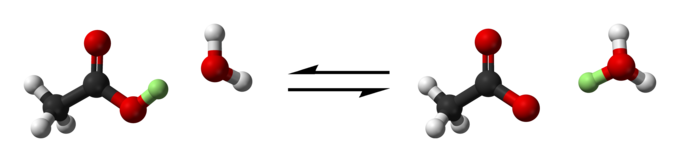## Specialized Equilibrium Constants

#### Learning Objective

• List the various special types of equilibrium constants

#### Key Points

• The self-ionization of water is the dissociation of water into a proton and a hydroxide ion. The expression Kw is defined as the product of the concentration of hydrogen ions and the concentration of hydroxide ions.
• The acid dissociation constant, Ka, measures the relative strength of an acid.
• The base dissociation constant, Kb, measures the relative strength of a base.

#### Terms

• self-ionizationThe process by which a water molecule donates a proton to a neighboring water molecule, yielding hydronium and hydroxide ions.
• dissociationThe process of breaking molecules apart into ions in solution.

Many reactions are so common or useful that they have their own special equilibrium constants.

## Self-Ionization of Water

The self-ionization, or autodissociation, of water is a reaction that occurs to a very small extent in neutral water. In this process, one molecule of water donates a proton to a neighboring water molecule, which yields hydronium and hydroxide ions.Autodissociation of waterA water molecule protonates a neighboring water molecule, yielding hydronium and hydroxide ions.

The equilibrium expression for this reaction is written as follows:

$K_W=[H^+][OH^-]=1.0\times 10^{-14}$

Note that because water is a liquid, it is omitted from this equilibrium expression. The value of the dissociation constant of water, KW, is $1.0\times 10^{-14}$. This will come into use later, in a future discussion on acids and bases.

## Acid Dissociation Constant, Ka

An acid dissociation constant, Ka, is the equilibrium constant for the dissociation of an acid in aqueous solution. The general form of the balanced equation is:

$HA(aq) \rightleftharpoons H^+(aq)+A^-(aq)$

HA is a generic acid that dissociates by splitting into A, known as the conjugate base of the acid, and a hydrogen ion, or proton, H+. As described previously, hydrogen ions actually exist as solvated hydronium ions in aqueous solutions. The equilibrium expression is given as:

$K_a=\frac{[H^+][A^-]}{[HA]}$

Recall that strong acids dissociate completely or almost completely into their ions. As such, strong acids will have large values of Ka that are greater than one, which indicates that the forward reaction of dissociation is strongly favored. Weak acids, on the other hand, will have small values of Ka that are less than one, indicating that the reverse reaction is strongly favored; weak acids dissociate only to a small extent. As such, Ka acts a relative indicator of acid strength.Acetic acid dissociationA ball-and-stick model of the dissociation of acetic acid to acetate. A water molecule is protonated to form a hydronium ion in the process. The acidic proton that is transferred from acetic acid to water is shown in green.

## Base Dissociation Constant, Kb

The base dissociation constant, Kb, is analogous to the acid dissociation constant. For a generalized reaction of a base, B, in water, we have:

$B(aq)+H_2O(l)\rightleftharpoons BH^+(aq)+OH^-(aq)$

The equilibrium expression, Kb, is given by:

$K_b=\frac{[BH^+][OH^-]}{[B]}$

As with the acid dissociation constant, large values of Kb are indicative of a stronger base, while small values of Kb are indicative of a weaker base.